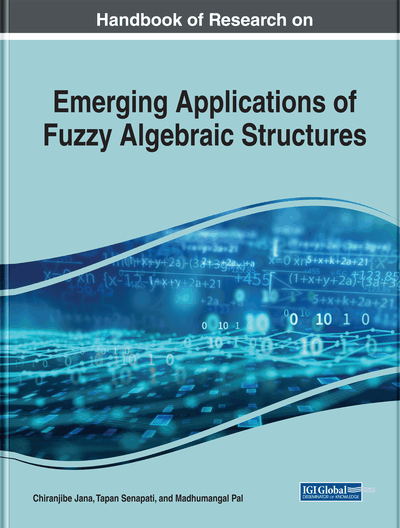Bipolar Fuzzy Structure of H-Ideals in BCI-Algebras

Tapan Senapati (Southwest University, China) and Guiyun Chen (Southwest University, China)
DOI: 10.4018/978-1-7998-0190-0.ch011
Available
\$37.50
No Current Special Offers

Abstract

In this chapter, the concepts of bipolar fuzzy H-ideals of BCI-algebras are introduced and their natures are investigated. Relations between bipolar fuzzy subalgebras, bipolar fuzzy ideals, and bipolar fuzzy H-ideals are discussed. Conditions for a bipolar fuzzy ideal to be a bipolar fuzzy H-ideal are provided. Some characterization theorems of bipolar fuzzy H-ideals are established. A bipolar fuzzy H-ideal is established by using a finite collection of H-ideals. The authors have shown that if every bipolar fuzzy H-ideal has the finite image, then every descending chain of H-ideals terminates at finite step.
Chapter Preview
Top

1. Introduction

Zhang (1994) initiated the concept of bipolar fuzzy sets (BFSs) as a generalization of fuzzy sets (Zadeh, 1965). In fuzzy sets membership degree range is [0,1]. In a BFS membership degree range is increased from the interval [0,1] to the interval [-1,1]. The membership degree 0 means that elements are irrelevant to the corresponding property, the membership degrees on (0,1] indicate that elements somewhat satisfy the property and the membership degrees on [-1,0] indicate that elements somewhat satisfy the implicit counter-property. This domain has recently motivated new research in several directions (Akram, 2011, Dubois, 2008, Zadrozny & Kacprzyk, 2012, Zhang & Zhang, 2004).

BCK-algebras and BCI-algebras are two important classes of logical algebras introduced by Imai & Iseki (1966). It is known that the class of BCK -algebra is a proper subclass of the class of BCI -algebras. Xi (1991) applied the concept of fuzzy sets to BCK-algebras. Jun (1993) applied it to BCI -algebras. Khalid and Ahmad (1999) introduced fuzzy H-ideals in BCI -algebras. Senapati et al. (2013, 2015) introduced (intuitionistic) fuzzy translations of (intuitionistic) fuzzy H-ideals in BCK/BCI-algebras and investigated their properties in details. The author (together with colleagues) have done lot of works on B/BG/G-algebras (Bhowmik et al, 2014, Senapati et al. 2012, 2014, 2015a, 2015b, 2015c, 2016) which is related to BCK/BCI -algebras.

Lee (2009) introduced the notion of bipolar fuzzy subalgebras and ideals in BCK/BCI -algebras. The concept of bipolar valued fuzzy translation and bipolar valued fuzzy S-extension of a bipolar valued fuzzy subalgebra in BCK/BCI -algebra was introduced by Jun et al.(2009). Lee et al. (2011) also extended this study to a-ideals of BCI -algebras.

Motivated by this, in this chapter, the notions of bipolar fuzzy H-ideals (BFHIs) of BCI-algebras is introduced and their properties are investigated. Relations among bipolar fuzzy subalgebras, bipolar fuzzy ideals and BFHIs are discussed. Conditions for a bipolar fuzzy ideal to be a BFHI are provided. Some characterization theorems of BFHIs are established. A BFHI is established by using a finite collection of H-ideals.

Top

2. Preliminaries

In this section, some elementary aspects that are necessary for this paper are included.

By a BCI-algebra we mean an algebra (X,*,0) of type (2,0) satisfying the following axioms for all x,y,z,X:

• 1.

((x*y)*(x*z))*(z*y)=0

• 2.

(x*(x*y))*y=0

• 3.

x*x=0

• 4.

x*y=0 and y*x=0 imply x=y.

Complete Chapter List

Search this Book:
Reset